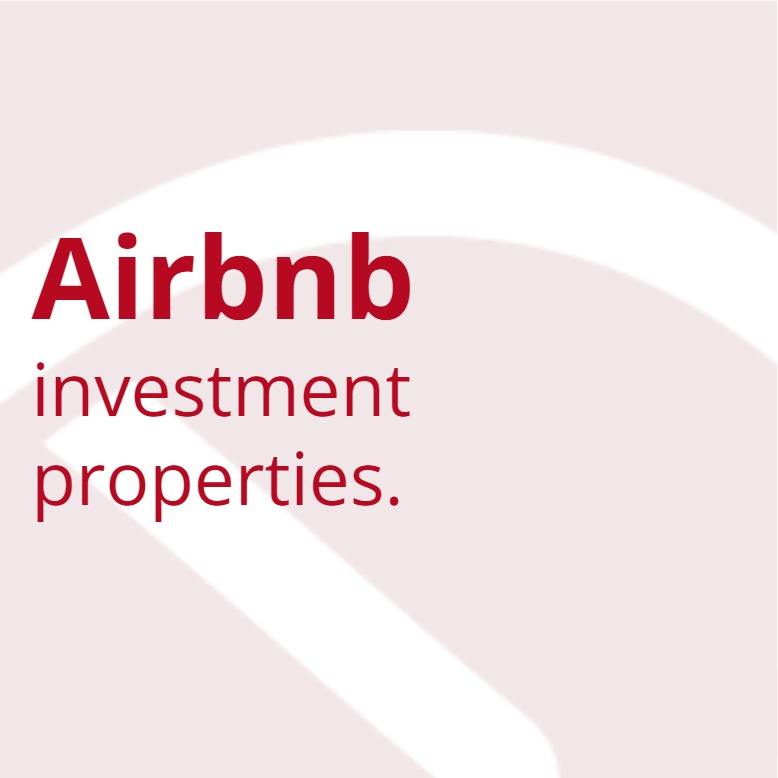### Magazinelast update Monday, April 24, 2017, keywords:, ,

In a discounted cash flow valuation, the cash flow is projected for each year into the future for a certain number of years, after which unique annual cash flows cannot be forecasted with reasonable accuracy. At that point, rather than attempting to forecast the varying cash flow for each individual year, one uses a single value representing the discounted value of all subsequent cash flows. This single value is referred to as the terminal value.

The terminal value can represent a large portion of the valuation. The terminal value of a piece of manufacturing equipment at the end of its useful life is its salvage value, typically less than 10% of the present value. In contrast, the terminal value associated with a business often is more than 50% of the total present value. For this reason, the terminal value calculation often is critical in performing a valuation. The terminal value can be calculated either based on the value if liquidated or based on the value of the firm as an ongoing concern.

#### Terminal Value if Liquidated

If the firm is to be liquidated, the liquidation value can be based on book value, salvage value, or break-up value, but liquidation value usually understates the terminal value of a healthy business. One must make assumptions about the salvage value of the assets and net working capital. The net working capital may have a certain recovery rate since it might not be readily liquidated at balance sheet values. In the pro forma projections, one often may assume that net working capital will grow at the same rate as cash flow. The terminal value if the firm is liquidated then is the sum of the discounted value of the cash flow, the recovered net working capital, and the salvage value of the long-term assets, including any tax benefits.

#### Terminal Value of the Ongoing Firm

For an ongoing firm, the terminal value may be determined by either using discounted cash flow (DCF) estimates or by using multiples from comparable firms.

For the DCF method, if the unlevered free cash flow is growing at a rate of  g  per year for a set number of years, the terminal value can be calculated by modeling the cash flow as a T-year growing perpetuity. At the end of T years, one can assume a different growth rate (possibly zero) or liquidation. If multiples from comparable firms are used, the price/earnings ratio, market/book values, or cash flow multiples are commonly used.

The unlevered terminal value is calculated using the return on assets (rA) as the discount rate. The levered terminal value is calculated using the weighted average cost of capital (WACC) as the discount rate.

#### Terminal Value of the Debt

The terminal value of debt or preferred stock is simply the projected book value of the debt or preferred stock in the year that the terminal value is being calculated.

#### Terminal Value of the Common Stock

The terminal value of the common stock is the total levered terminal value less the terminal value of the debt, less the terminal value of the preferred stock (adding in the amount from any warrants that are exercised at their exercise price), plus the cash gained from the exercise of any common and preferred warrants.

Page keywords: ,

What is next? Go ahead and share it!

check this out, Terminal Value

Want more content ? Subscribe PaperFree  Magazine!

We will send you an email with the top content on this subject: , .

Invest in long-life, high-quality assets around the world.